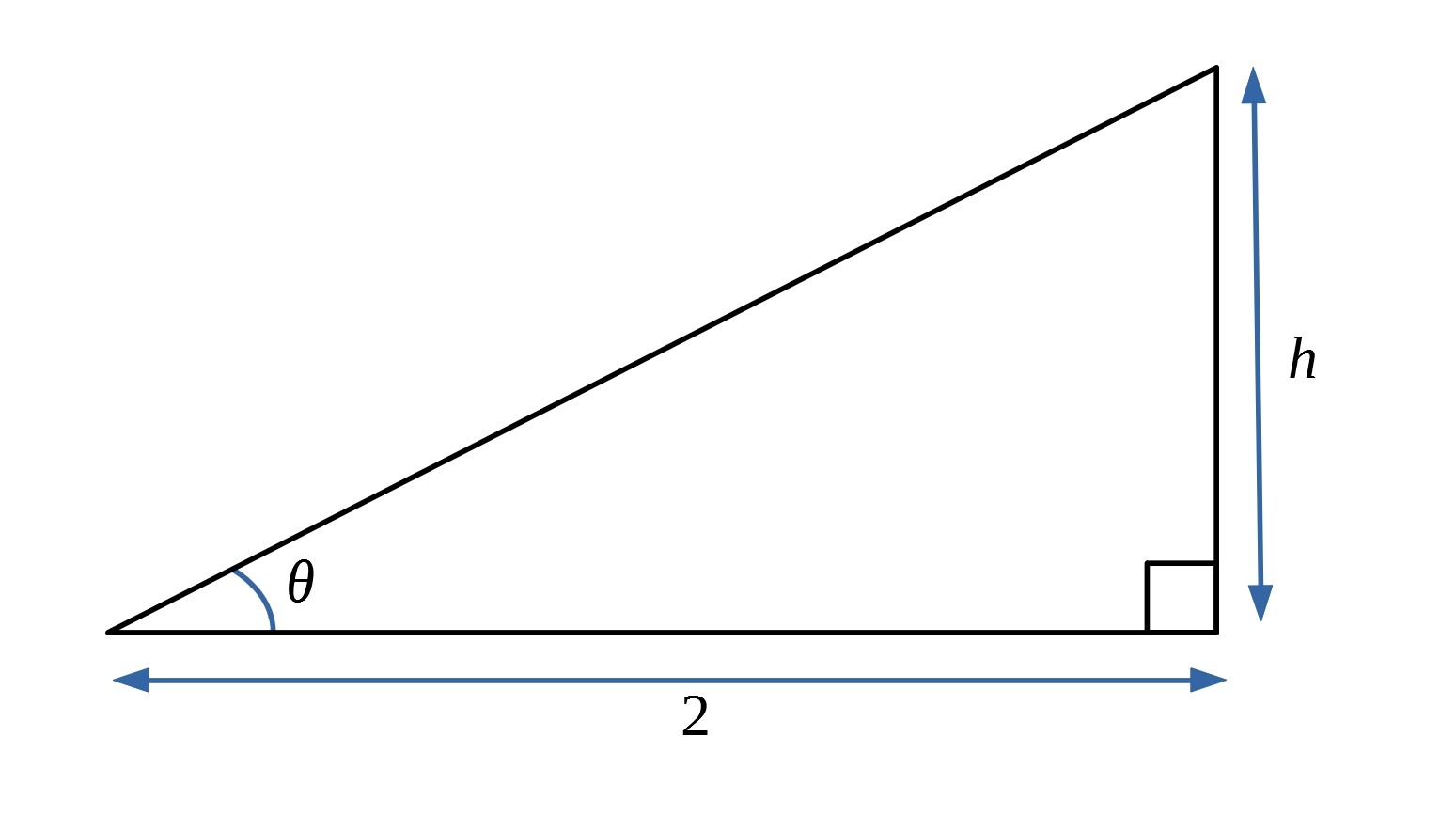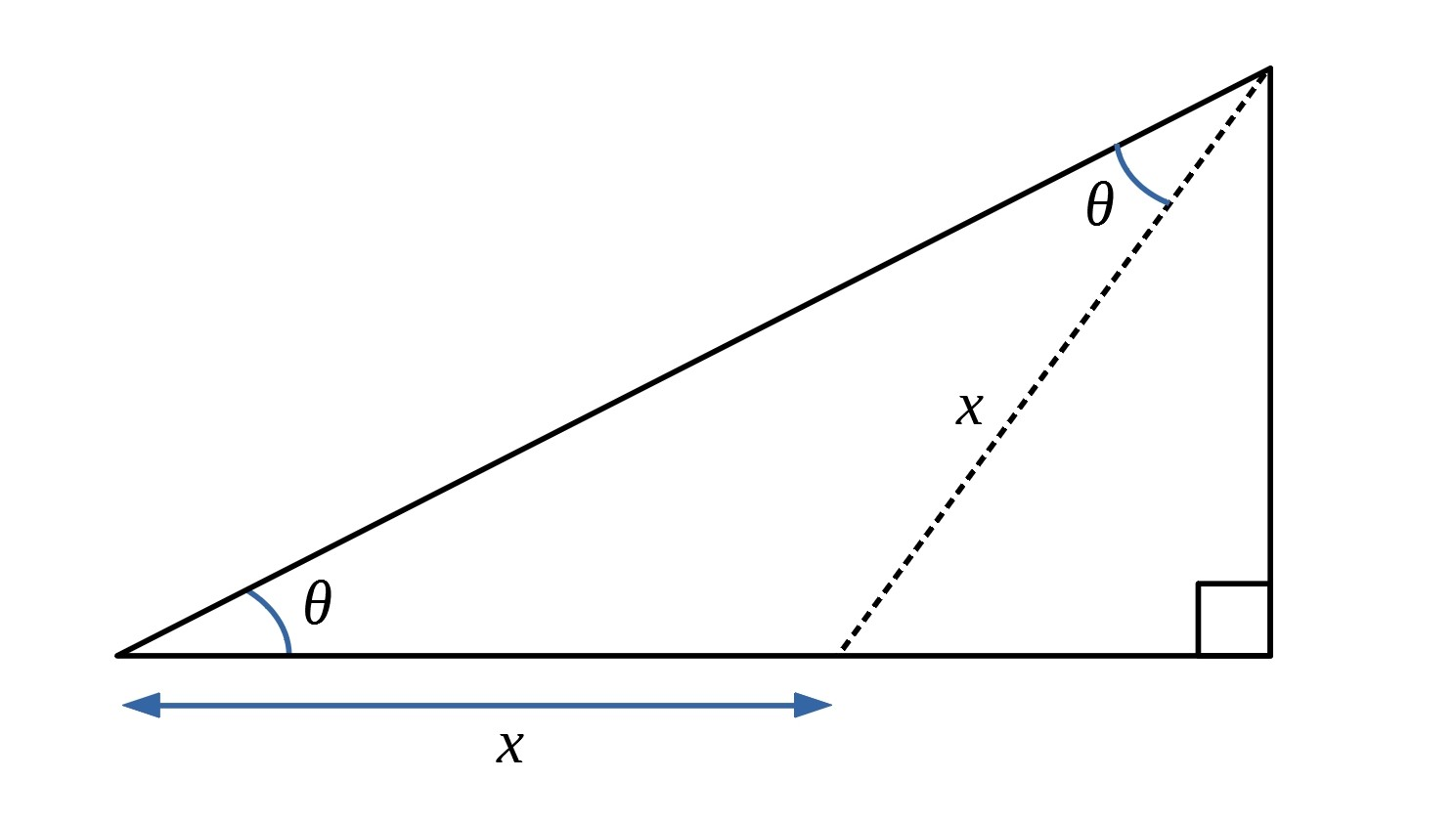#### You may also like### Shape and Territory

If for any triangle ABC tan(A - B) + tan(B - C) + tan(C - A) = 0 what can you say about the triangle?### Why Stop at Three by One

Beautiful mathematics. Two 18 year old students gave eight different proofs of one result then generalised it from the 3 by 1 case to the n by 1 case and proved the general result.### Reflect Again

Follow hints to investigate the matrix which gives a reflection of the plane in the line y=tanx. Show that the combination of two reflections in intersecting lines is a rotation.

# T for Tan

##### Age 16 to 18Challenge Level

Consider a right angled triangle with an acute angle of $\theta$.  Let the base of the triangle be of length 2.Find the height of the triangle in terms of $t$, where $t=\tan \theta$.

Now imagine a line in the triangle which forms an isosceles triangle with two angles equal to $\theta$.Use this diagram to prove the double angle formula, where $t=\tan \theta$: $$\tan2\theta = {2t\over {1-t^2}}, \quad \sin2\theta = {2t\over {1+t^2}},\quad \cos2\theta = {{1-t^2}\over {1+t^2}}$$